Скачать презентацию RT-LAB Solution for Real-Time Applications Hardware-In-Loop introduction 3 20 09

2e904b54fcf1b8c968aa7a7a3b188c94.ppt

• Количество слайдов: 35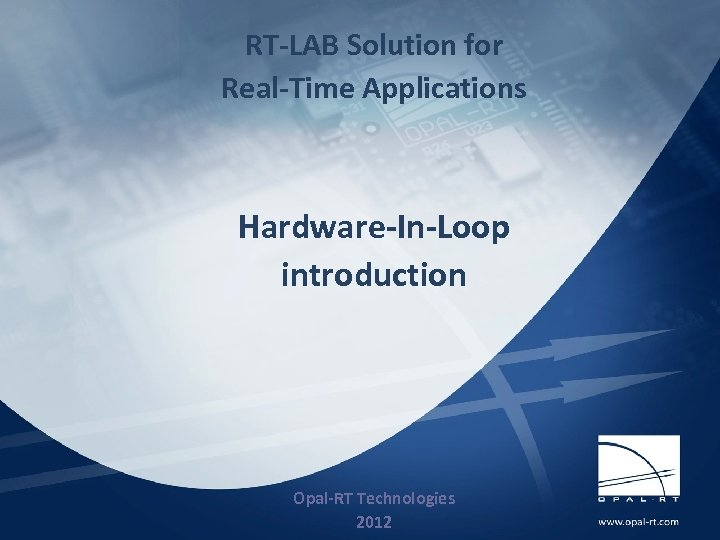RT-LAB Solution for Real-Time Applications Hardware-In-Loop introduction 3/20/09 Opal-RT Technologies 2012 1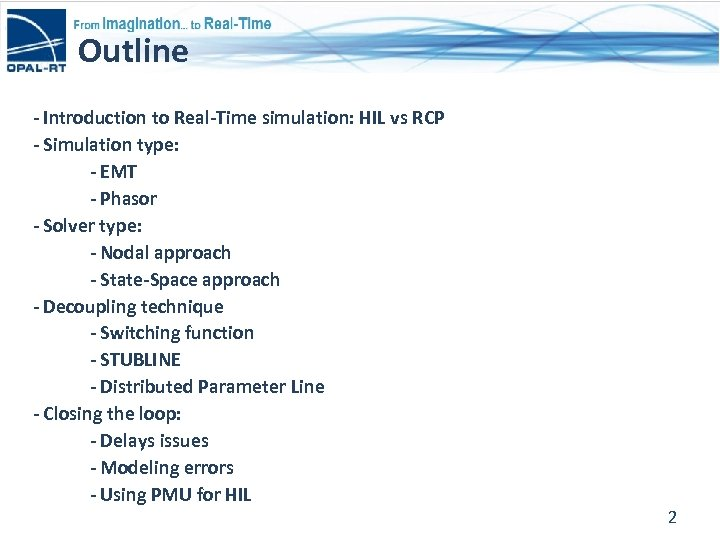Outline - Introduction to Real-Time simulation: HIL vs RCP - Simulation type: - EMT - Phasor - Solver type: - Nodal approach - State-Space approach - Decoupling technique - Switching function - STUBLINE - Distributed Parameter Line - Closing the loop: - Delays issues - Modeling errors - Using PMU for HIL 2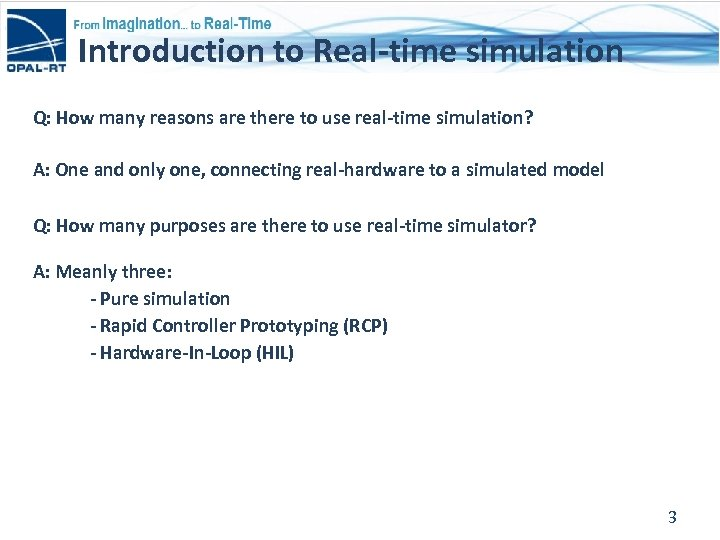Introduction to Real-time simulation Q: How many reasons are there to use real-time simulation? A: One and only one, connecting real-hardware to a simulated model Q: How many purposes are there to use real-time simulator? A: Meanly three: - Pure simulation - Rapid Controller Prototyping (RCP) - Hardware-In-Loop (HIL) 3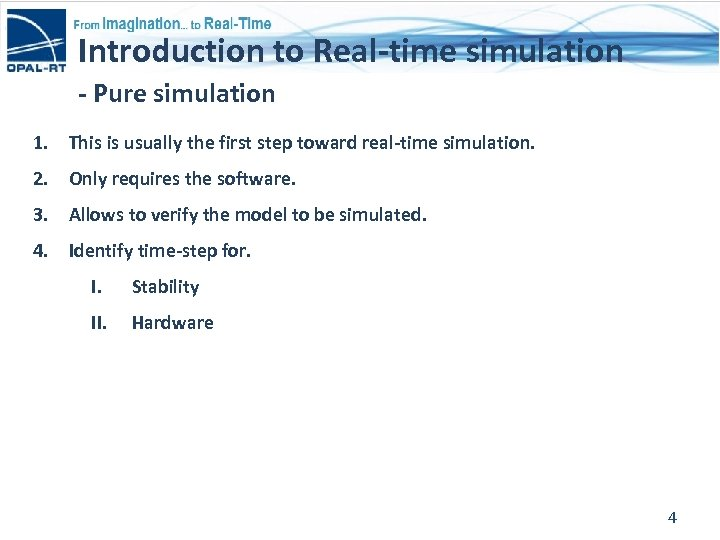Introduction to Real-time simulation - Pure simulation 1. This is usually the first step toward real-time simulation. 2. Only requires the software. 3. Allows to verify the model to be simulated. 4. Identify time-step for. I. Stability II. Hardware 4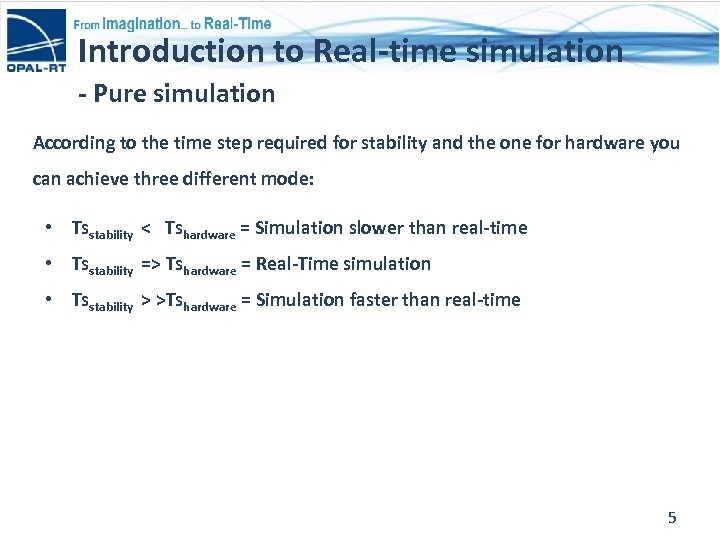Introduction to Real-time simulation - Pure simulation According to the time step required for stability and the one for hardware you can achieve three different mode: • Tsstability < Tshardware = Simulation slower than real-time • Tsstability => Tshardware = Real-Time simulation • Tsstability > >Tshardware = Simulation faster than real-time 5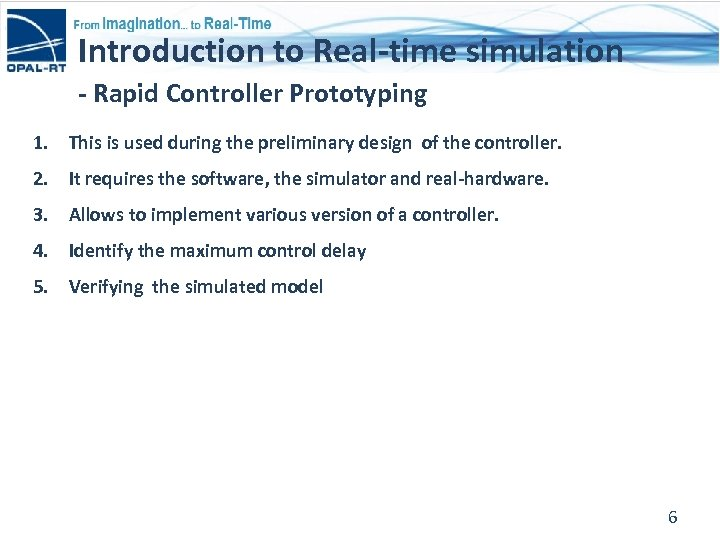Introduction to Real-time simulation - Rapid Controller Prototyping 1. This is used during the preliminary design of the controller. 2. It requires the software, the simulator and real-hardware. 3. Allows to implement various version of a controller. 4. Identify the maximum control delay 5. Verifying the simulated model 6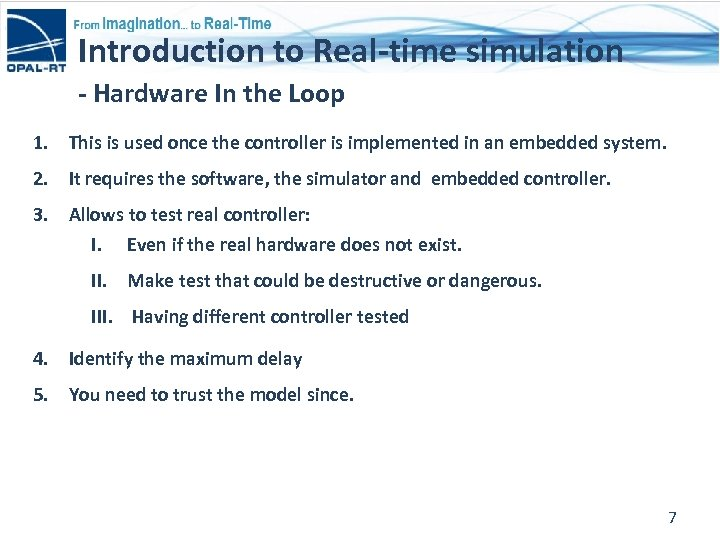Introduction to Real-time simulation - Hardware In the Loop 1. This is used once the controller is implemented in an embedded system. 2. It requires the software, the simulator and embedded controller. 3. Allows to test real controller: I. Even if the real hardware does not exist. II. Make test that could be destructive or dangerous. III. Having different controller tested 4. Identify the maximum delay 5. You need to trust the model since. 7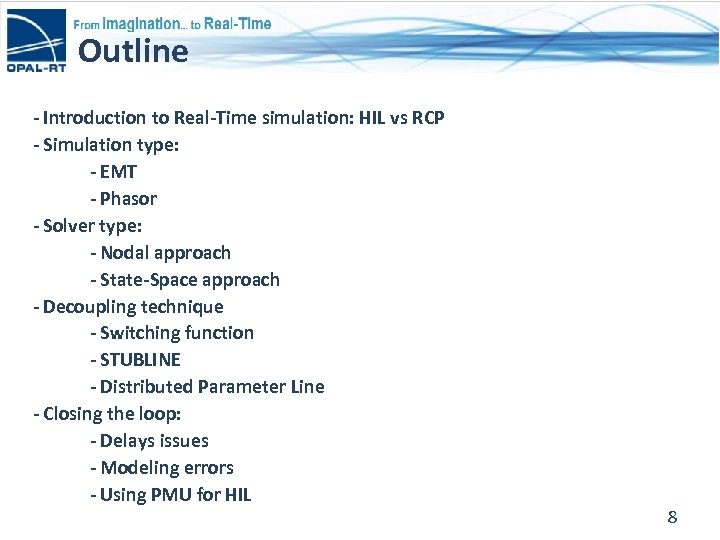Outline - Introduction to Real-Time simulation: HIL vs RCP - Simulation type: - EMT - Phasor - Solver type: - Nodal approach - State-Space approach - Decoupling technique - Switching function - STUBLINE - Distributed Parameter Line - Closing the loop: - Delays issues - Modeling errors - Using PMU for HIL 8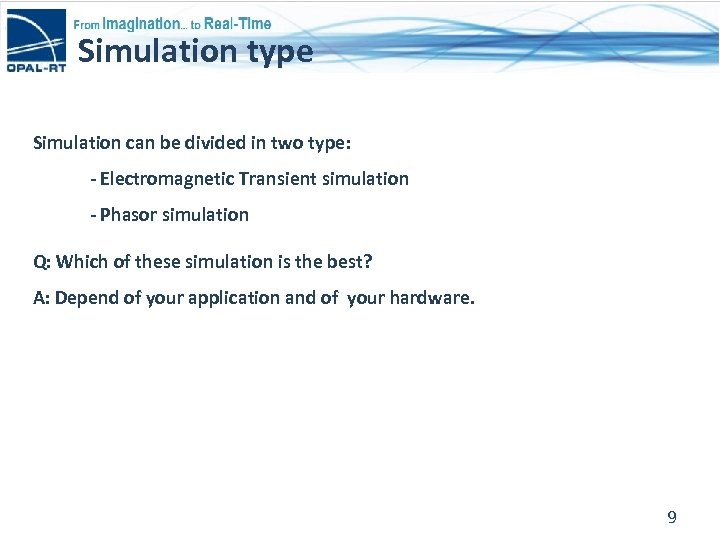Simulation type Simulation can be divided in two type: - Electromagnetic Transient simulation - Phasor simulation Q: Which of these simulation is the best? A: Depend of your application and of your hardware. 9Simulation type -Phasor simulation - Uses a large time step (few millisecond). - Used for very large model. - Only simulate the positive sequence. - General application are: - Integration of distributed energy resources and load models to the simulator - Operator Training Simulator (OTS) - Dynamic Security Assessment (DSA) - Test, tune, and optimize setting of control devices - Test SCADA systems with PMU measurements - Test and adjustment local control systems such as transformer tapchanger, capacitor banks 10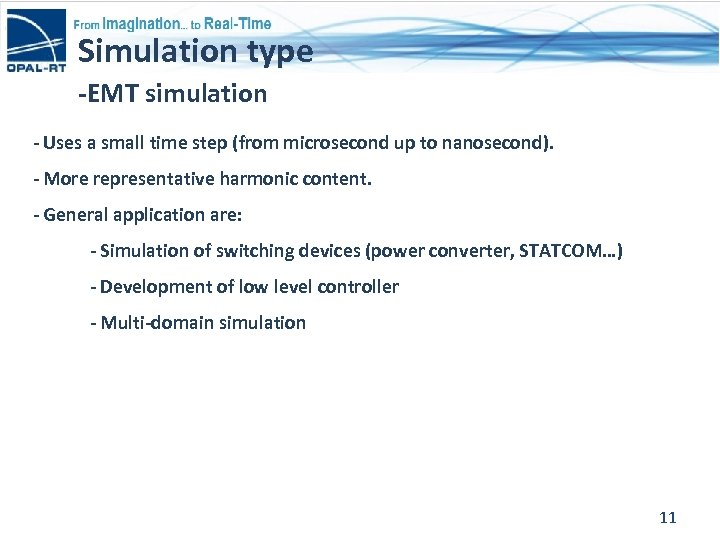Simulation type -EMT simulation - Uses a small time step (from microsecond up to nanosecond). - More representative harmonic content. - General application are: - Simulation of switching devices (power converter, STATCOM…) - Development of low level controller - Multi-domain simulation 11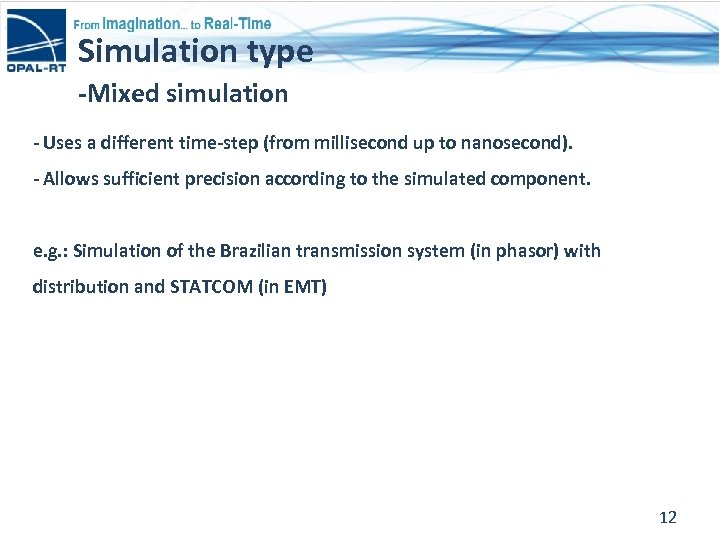Simulation type -Mixed simulation - Uses a different time-step (from millisecond up to nanosecond). - Allows sufficient precision according to the simulated component. e. g. : Simulation of the Brazilian transmission system (in phasor) with distribution and STATCOM (in EMT) 12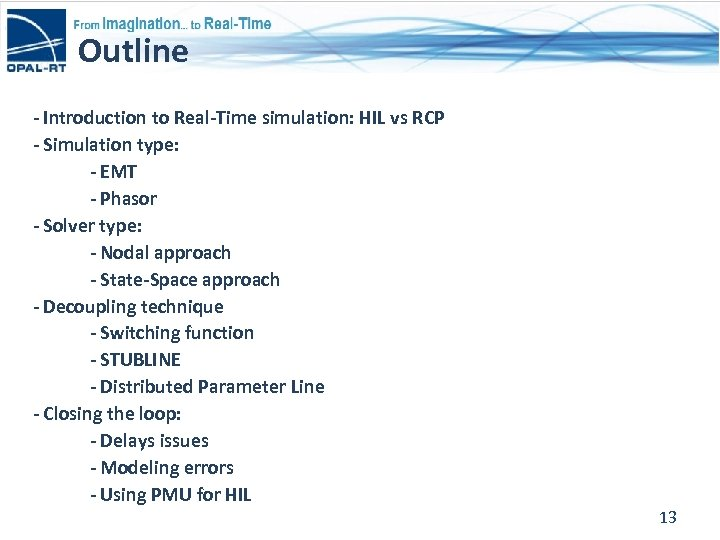Outline - Introduction to Real-Time simulation: HIL vs RCP - Simulation type: - EMT - Phasor - Solver type: - Nodal approach - State-Space approach - Decoupling technique - Switching function - STUBLINE - Distributed Parameter Line - Closing the loop: - Delays issues - Modeling errors - Using PMU for HIL 13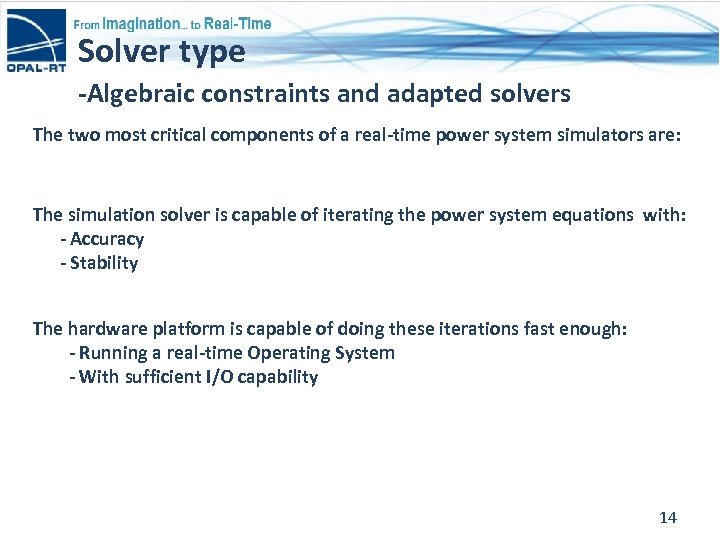Solver type -Algebraic constraints and adapted solvers The two most critical components of a real-time power system simulators are: The simulation solver is capable of iterating the power system equations with: - Accuracy - Stability The hardware platform is capable of doing these iterations fast enough: - Running a real-time Operating System - With sufficient I/O capability 14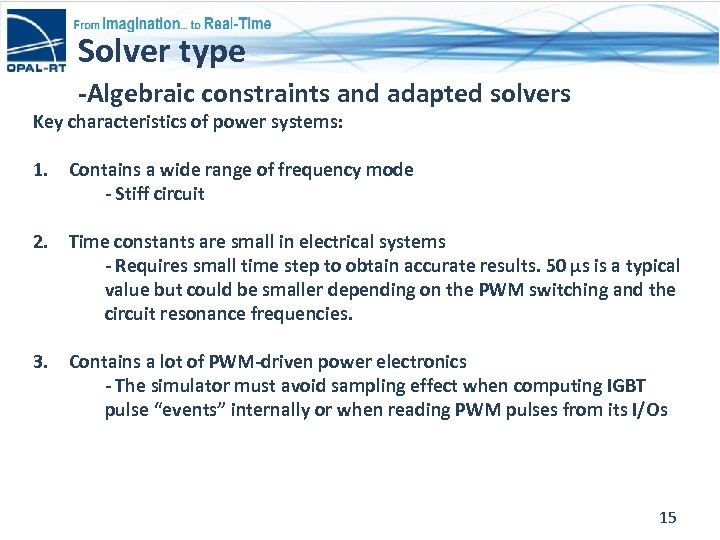Solver type -Algebraic constraints and adapted solvers Key characteristics of power systems: 1. Contains a wide range of frequency mode - Stiff circuit 2. Time constants are small in electrical systems - Requires small time step to obtain accurate results. 50 µs is a typical value but could be smaller depending on the PWM switching and the circuit resonance frequencies. 3. Contains a lot of PWM-driven power electronics - The simulator must avoid sampling effect when computing IGBT pulse “events” internally or when reading PWM pulses from its I/Os 15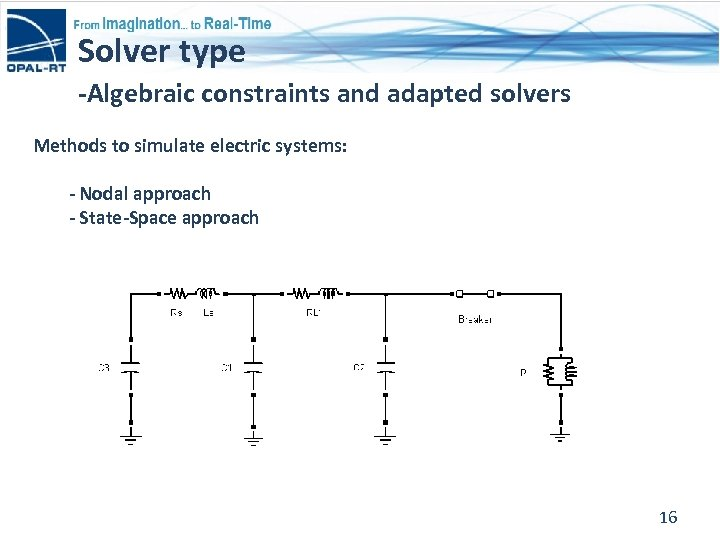Solver type -Algebraic constraints and adapted solvers Methods to simulate electric systems: - Nodal approach - State-Space approach 16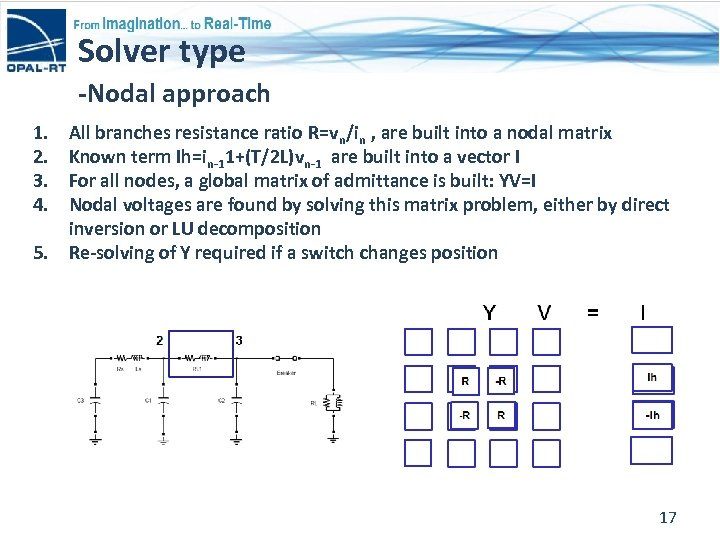Solver type -Nodal approach 1. 2. 3. 4. 5. All branches resistance ratio R=vn/in , are built into a nodal matrix Known term Ih=in-11+(T/2 L)vn-1 are built into a vector I For all nodes, a global matrix of admittance is built: YV=I Nodal voltages are found by solving this matrix problem, either by direct inversion or LU decomposition Re-solving of Y required if a switch changes position 17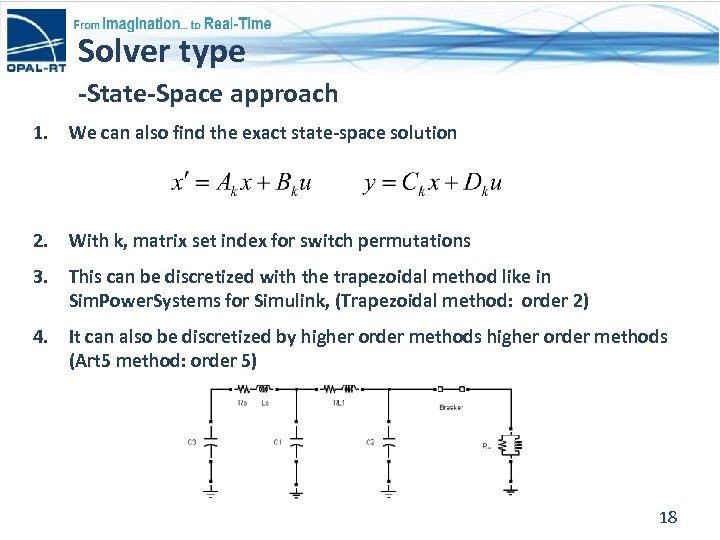Solver type -State-Space approach 1. We can also find the exact state-space solution 2. With k, matrix set index for switch permutations 3. This can be discretized with the trapezoidal method like in Sim. Power. Systems for Simulink, (Trapezoidal method: order 2) 4. It can also be discretized by higher order methods (Art 5 method: order 5) 18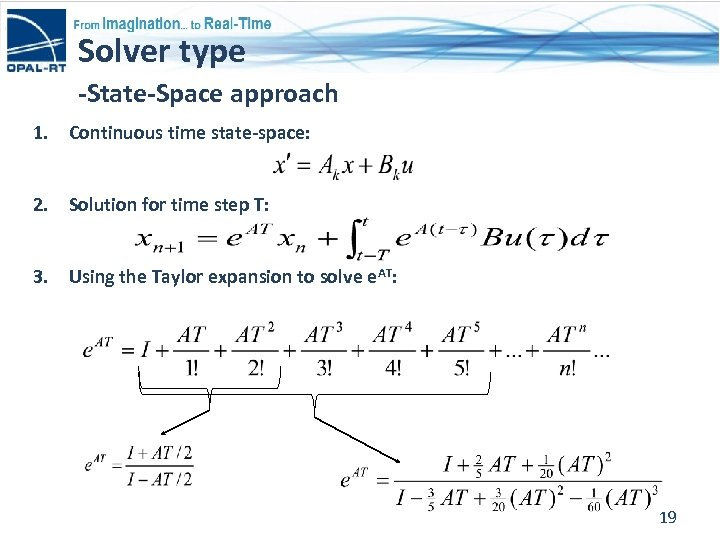Solver type -State-Space approach 1. Continuous time state-space: 2. Solution for time step T: 3. Using the Taylor expansion to solve e. AT: 19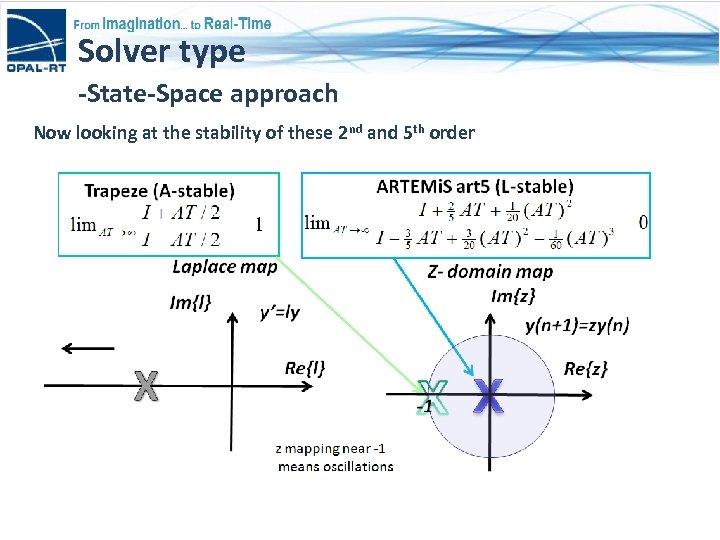Solver type -State-Space approach Now looking at the stability of these 2 nd and 5 th order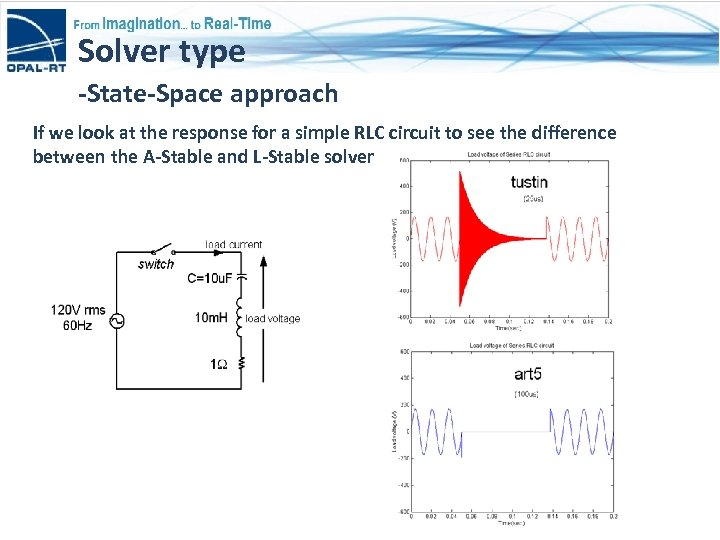Solver type -State-Space approach If we look at the response for a simple RLC circuit to see the difference between the A-Stable and L-Stable solver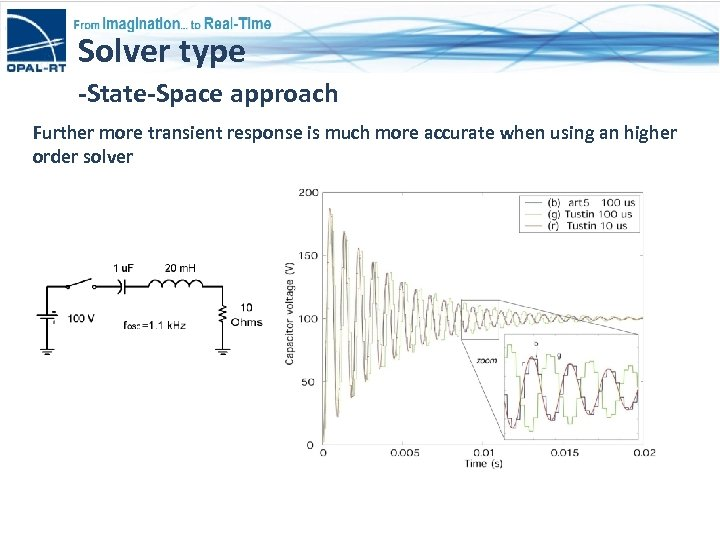Solver type -State-Space approach Further more transient response is much more accurate when using an higher order solver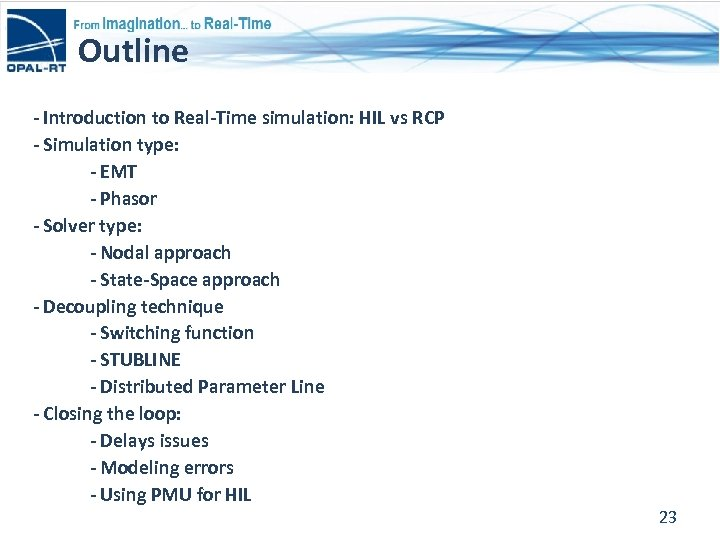Outline - Introduction to Real-Time simulation: HIL vs RCP - Simulation type: - EMT - Phasor - Solver type: - Nodal approach - State-Space approach - Decoupling technique - Switching function - STUBLINE - Distributed Parameter Line - Closing the loop: - Delays issues - Modeling errors - Using PMU for HIL 23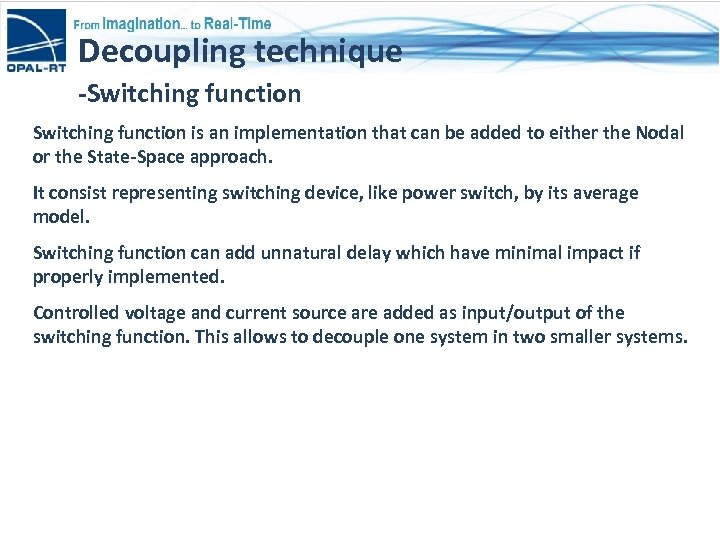Decoupling technique -Switching function is an implementation that can be added to either the Nodal or the State-Space approach. It consist representing switching device, like power switch, by its average model. Switching function can add unnatural delay which have minimal impact if properly implemented. Controlled voltage and current source are added as input/output of the switching function. This allows to decouple one system in two smaller systems.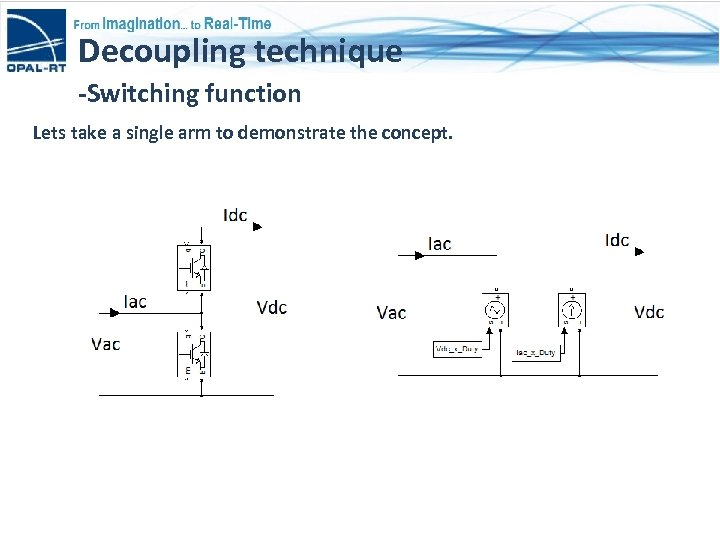Decoupling technique -Switching function Lets take a single arm to demonstrate the concept.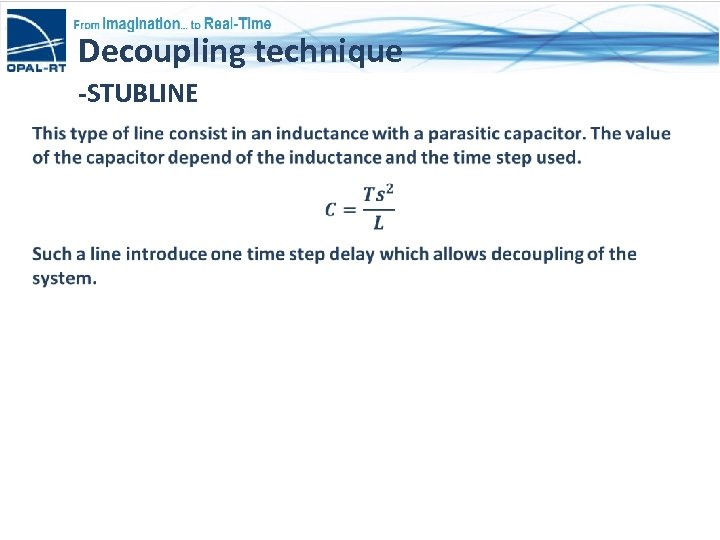Decoupling technique -STUBLINE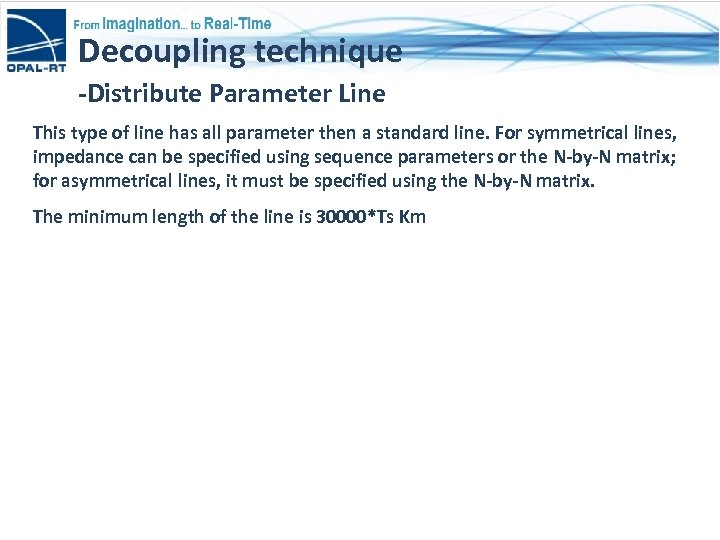Decoupling technique -Distribute Parameter Line This type of line has all parameter then a standard line. For symmetrical lines, impedance can be specified using sequence parameters or the N-by-N matrix; for asymmetrical lines, it must be specified using the N-by-N matrix. The minimum length of the line is 30000*Ts Km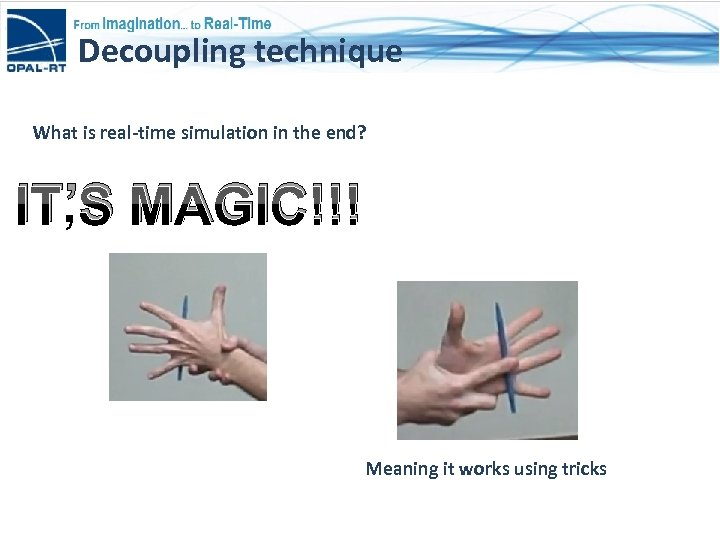Decoupling technique What is real-time simulation in the end? IT’S MAGIC!!! Meaning it works using tricks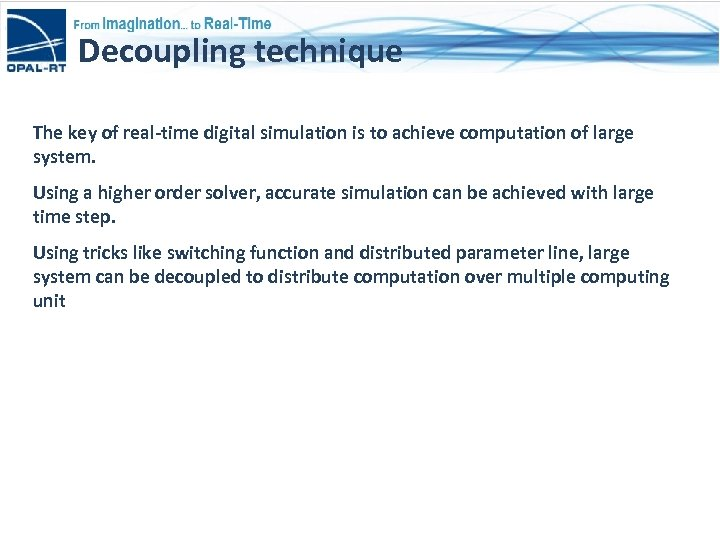Decoupling technique The key of real-time digital simulation is to achieve computation of large system. Using a higher order solver, accurate simulation can be achieved with large time step. Using tricks like switching function and distributed parameter line, large system can be decoupled to distribute computation over multiple computing unit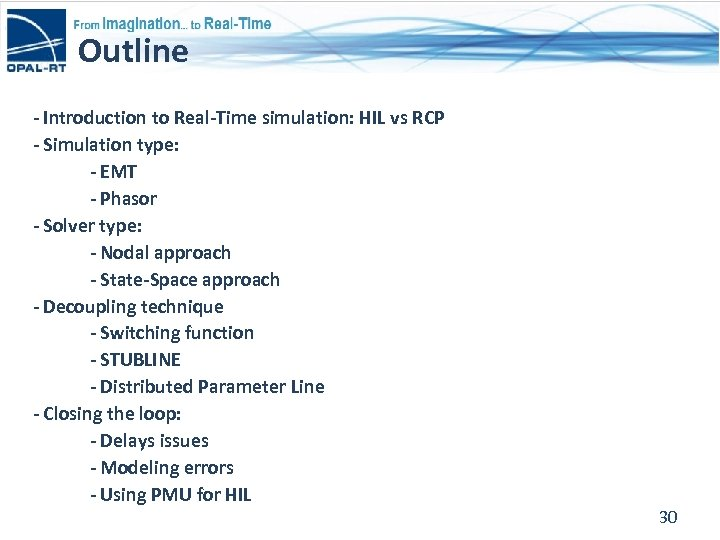Outline - Introduction to Real-Time simulation: HIL vs RCP - Simulation type: - EMT - Phasor - Solver type: - Nodal approach - State-Space approach - Decoupling technique - Switching function - STUBLINE - Distributed Parameter Line - Closing the loop: - Delays issues - Modeling errors - Using PMU for HIL 30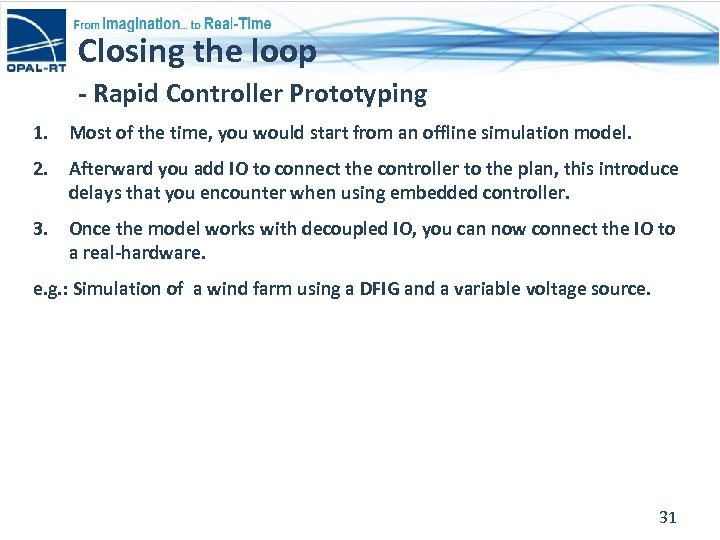Closing the loop - Rapid Controller Prototyping 1. Most of the time, you would start from an offline simulation model. 2. Afterward you add IO to connect the controller to the plan, this introduce delays that you encounter when using embedded controller. 3. Once the model works with decoupled IO, you can now connect the IO to a real-hardware. e. g. : Simulation of a wind farm using a DFIG and a variable voltage source. 31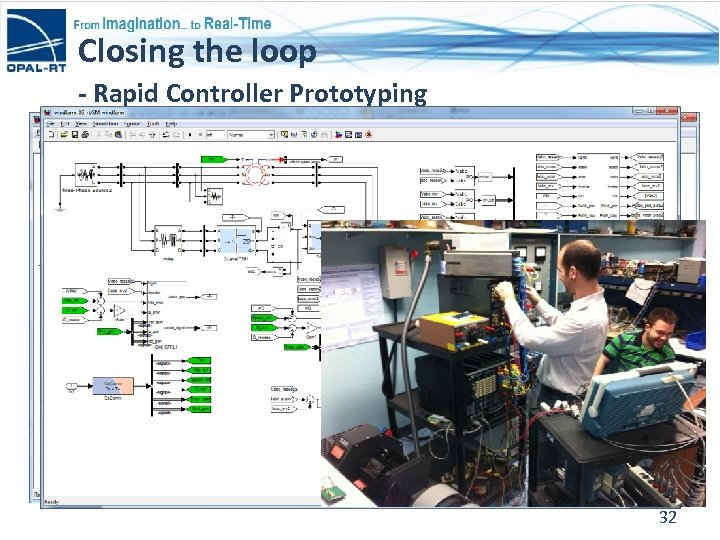Closing the loop - Rapid Controller Prototyping 32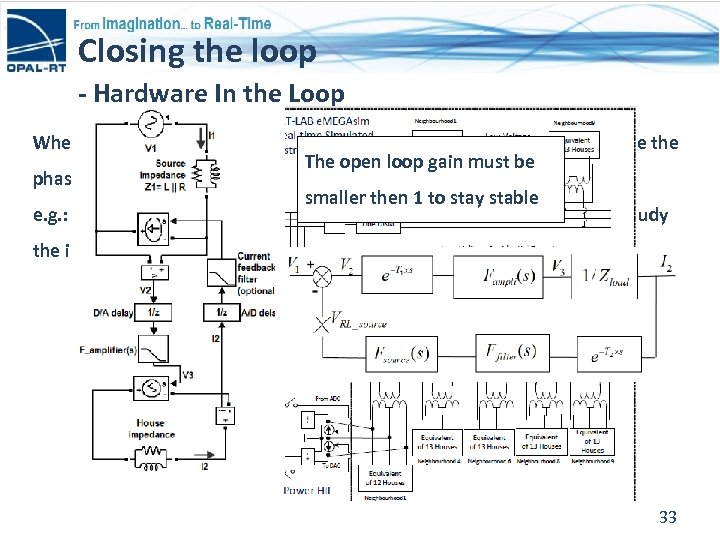Closing the loop - Hardware In the Loop When simulated model is coupled using power amplifier delay can change the The open loop gain must be phase of the signal and cause instability. smaller then 1 to stay stable e. g. : A simulated network is coupled with a real smart house using to study the impact of the smart house on the network. 33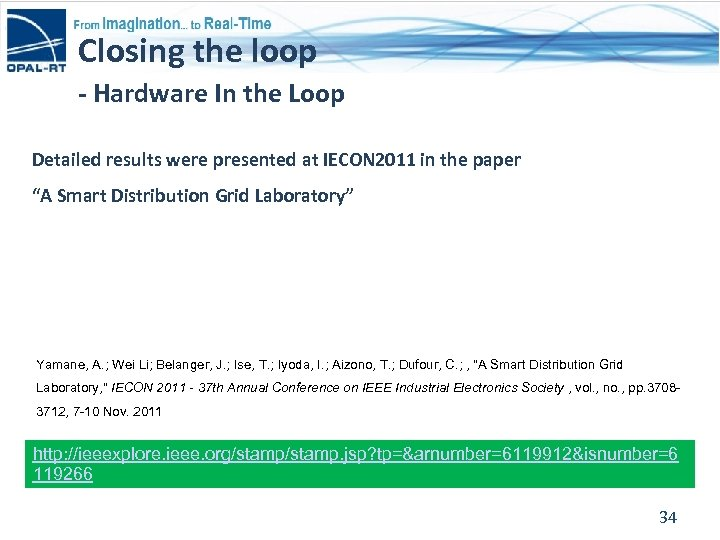Closing the loop - Hardware In the Loop Detailed results were presented at IECON 2011 in the paper “A Smart Distribution Grid Laboratory” Yamane, A. ; Wei Li; Belanger, J. ; Ise, T. ; Iyoda, I. ; Aizono, T. ; Dufour, C. ; , "A Smart Distribution Grid Laboratory, " IECON 2011 - 37 th Annual Conference on IEEE Industrial Electronics Society , vol. , no. , pp. 37083712, 7 -10 Nov. 2011 http: //ieeexplore. ieee. org/stamp. jsp? tp=&arnumber=6119912&isnumber=6 119266 34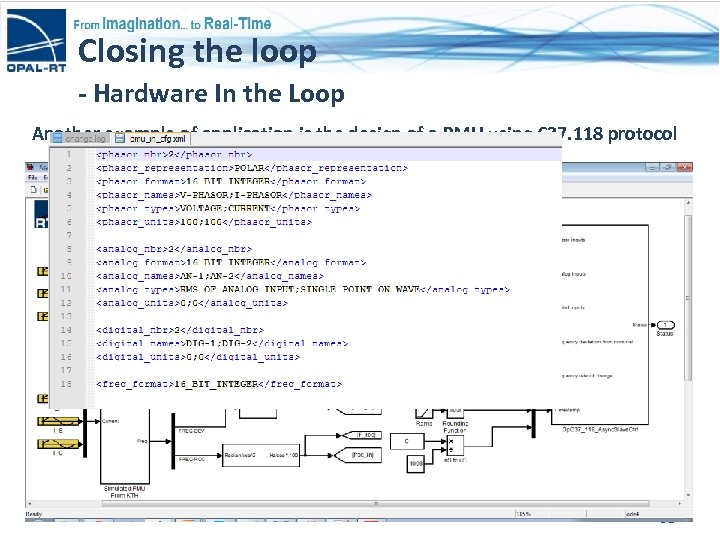Closing the loop - Hardware In the Loop Another example of application is the design of a PMU using C 37. 118 protocol 35